Next: 5.3.3 Direction Vector Calculation Up: 5.3 Generation of Random Previous: 5.3.1 Power Cosine Distribution

## 5.3.2 Coned Cosine Distribution

Next, the distribution introduced in (2.17) is considered, for which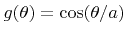for all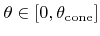. Hence, all angles are within a cone with apex angle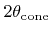. This is the reason for the name of the distribution used in this work.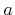and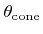are related by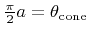. To restrict the emission of particles to one hemisphere,must satisfy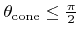, which is equivalent to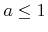. The probability density of the polar angle is given by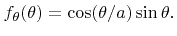(5.40)

The corresponding cumulative distribution function is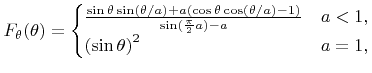(5.41)

Since it is not possible to calculate an explicit expression for the inverse function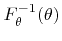, which is useful for the inverse method, the rejection technique is chosen instead . For the rejection method an instrumental distribution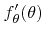is necessary, which is an upper bound approximation of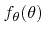, and which leads to an invertable cumulative distribution function.

Using the inequalities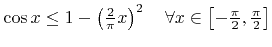(5.42)

and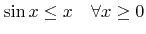(5.43)

(see Inequality 1 and Inequality 2 in Appendix C) such an instrumental probability density function for (5.40) is given by(5.44)

The corresponding cumulative distribution function is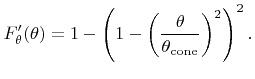(5.45)

According to the rejection method a random polar angle following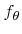can be generated by feeding the inverse of the instrumental cumulative distribution function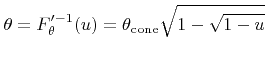(5.46)

with uniformly distributed random variates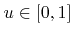and accepting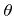, if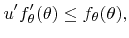(5.47)

where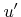denotes another uniformly distributed variate on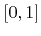. Algorithm 5.3 summarizes the entire procedure for determining random polar angles, which are distributed according to the coned cosine distribution.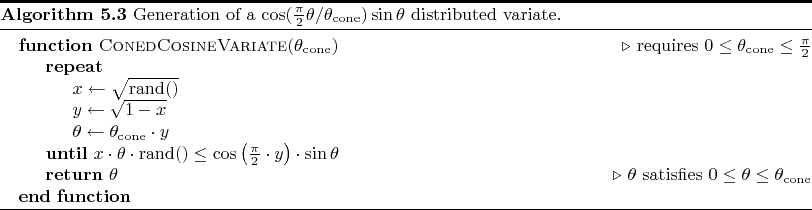The efficiencyof rejection sampling for this case, thus the fraction of successful attempts satisfying (5.47), is given by(5.48)

The presented algorithm shows a very high efficiency, since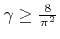holds for all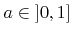(see Inequality 3 in Appendix C), which corresponds to a success rate of approximately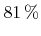in the worst case.Next: 5.3.3 Direction Vector Calculation Up: 5.3 Generation of Random Previous: 5.3.1 Power Cosine Distribution

Otmar Ertl: Numerical Methods for Topography Simulation